# Population Analysis

Analysis and Visualization of population with Python

Diwash Shrestha https://diwashrestha.com.np
06-23-2017

Today I am writing about Population though my last two posts were also about population.But, today in this post I will analyse the data and make some visuals using the data and find some answers. In this blog, I am going to use the dataset from the UN databank (Link:http://data.un.org/). This dataset is about the world population from 1950 to 2015. It has the population data of all the Continents, Country. I am using python to read this dataset and have a look in the CSV file. I am using Numpy for analysis or for arithmetic and Matplotlib for visualization of data and Jupyter Notebook as my Python workspace. I am using only the contents of 6 Continents(#Note: exclude Antarctica there is no permanent settlement) and population of the world for analysis and following are the code I wrote for it.


#Importing required python packages NUMPY and MATPLOTLIB
import numpy as np
import matplotlib.pyplot as plt

#using python to load the csv file
f=open("population data.csv","r")
rows = data.split('\n')
final_data =[]

In the following code, I used the function “region” to get the data of continent according to their name by searching in the list called “rows” and another function “Interize” to convert the string value in the list to Integer.


#Function to get the data from CSV file using the parameter name to search data using loop in given list of data

def region(R_name):
final_data = [] # a list which will hold the data taken from rows list for some time
for pop_data in rows: # loop to search the row in list rows, we split the data after ","  and append in final_data
pop = pop_data.split(',')
final_data.append(pop)
Region_data = [] # Declaring new list which will
for datum in final_data:
if R_name in datum:
Region_data.append(datum)
Region_data = Region_data[1:] # Region_data has list in list so to have only one list data
return Region_data
World = region(R_name="WORLD")
Africa = region(R_name="AFRICA")
Asia  = region(R_name="ASIA")
Europe = region(R_name="EUROPE")
Latinamerica  = region(R_name="LATIN AMERICA AND THE CARIBBEAN")
NorthAmerica = region(R_name="NORTHERN AMERICA")
Oceania  = region(R_name="OCEANIA")

#function to make the string data in list to Integer
def Interize(List):
lone_list =[]
for data in List:
lone_list.append(int(data))
return lone_list
Africa = Interize(Africa)
Asia = Interize(Asia)
Europe =Interize(Europe)
Latinamerica =Interize(Latinamerica)
NorthAmerica =Interize(NorthAmerica)
Oceania =Interize(Oceania)
World = Interize(World)

print ("----World-----")
print (World)
print ("-----AFRICA------")
print (Africa)
print ("----ASIA-----")
print (Asia)
print ("----Europe----")
print (Europe)
print ("----Latin America-----")
print (Latinamerica)
print ("----North America-----")
print (NorthAmerica)
print ("-----Oceania------")
print (Oceania)

----World-----
[2525779, 2761651, 3026003, 3329122, 3691173, 4071020, 4449049, 4863602, 5320817, 5741822, 6127700, 6514095, 6916183, 7324782]
-----AFRICA------
[228827, 253988, 285270, 322581, 366475, 417413, 478459, 549846, 629987, 716505, 808304, 911528, 1031084, 1166239]
----ASIA-----
[1395749, 1537568, 1694650, 1881423, 2128631, 2387024, 2634161, 2907535, 3213123, 3482719, 3717372, 3942882, 4165440, 4384844]
----Europe----
[549043, 576912, 605517, 635004, 657369, 677662, 694510, 709189, 723248, 729743, 729105, 732970, 740308, 743123]
----Latin America-----
[167869, 192271, 220439, 253153, 287588, 324746, 364150, 404329, 445203, 486345, 526278, 562546, 596191, 630089]
----North America-----
[171615, 186744, 204352, 219467, 231429, 242685, 254800, 267831, 282286, 297458, 315417, 330546, 346501, 361128]
-----Oceania------
[12675, 14167, 15775, 17494, 19681, 21492, 22968, 24872, 26969, 29052, 31224, 33623, 36659, 39359]

#making a Year list which will be used to visualize in graphs
year = np.arange(1950,2020,5)
print ("year"),
print (year)

year
[1950 1955 1960 1965 1970 1975 1980 1985 1990 1995 2000 2005 2010 2015]

#using .plot to plot a graph using the population value and year value
#
plt.figure(figsize=(15,8.5))
#a function to plot the graph which has two argument time_line as x and region as y
def ploting(time_line,Region_name,mark):
plt.plot(time_line,Region_name,marker=mark)

ploting(year,Asia,"*")
ploting(year,Africa,"o")
ploting(year,Europe,"*")
ploting(year,NorthAmerica,"*")
ploting(year,Latinamerica,"*")
ploting(year,Oceania,"o")
labels = 'Asia','Africa', 'Europe','NorthAmerica' ,'Latinamerica','Oceania'
plt.xlabel("Year",size=12,color="green")
plt.ylabel("Population in thousand",size=12,color="blue")
plt.legend(labels, loc="best")
plt.title("Population Distribution Acc. to Continents over period of time",color="red",size=15)
plt.show()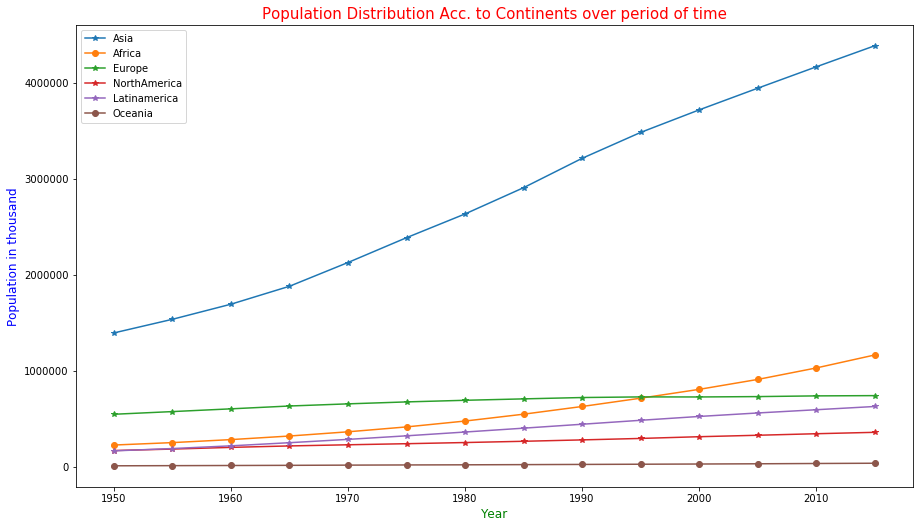In the above plot, we can see the size of the population of continents from 1950 to 2015 using the legend and graphs plot. This plot is using Year as X-axis and Population in Thousand as Y-axis. We can see all the continent’s population is increasing but Europe’s is starting to decrease from the year 1995. It may seem the population of Oceania region is Zero but not as the population of Australia is very small and this graph is using population in thousand bases so it seems Oceania is zero.


#Making pizza chart or pac_man chart
plt.figure(figsize=(15, 7.5))
labels = 'Asia', 'Africa', 'Europe', 'Latinamerica','NorthAmerica','Oceania'
pop_sizes_2015 = [4384844,1166239, 743123, 630089,361128,39359 ]
colors = ['brown','yellow' , 'coral', 'lightskyblue','green','red']
explode = (0.1, 0.1, 0.1, 0.1,0.1,0.2)  #exploding the slice of pizza
plt.legend(labels, loc="best")
plt.axis('equal')
plt.title("Pie-Chart for the world population distribution 2015 Acc. Continent",color="red",size= 15)
plt.show()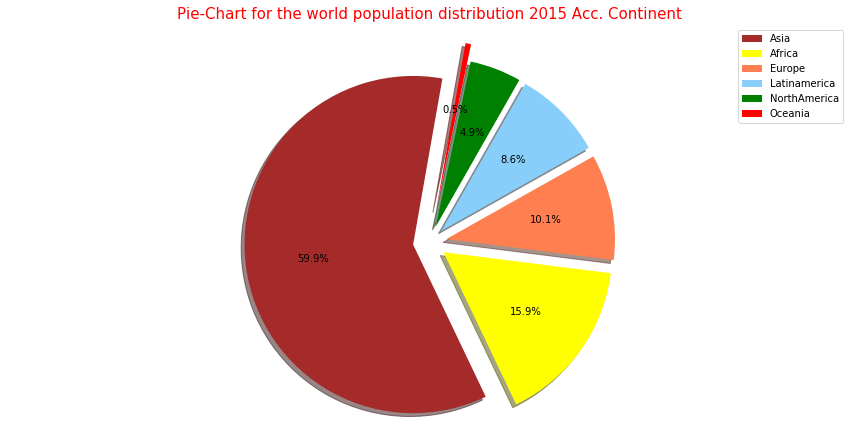This Pie-Chart shows us the population size of every continent in the year 2015. It shows us the population of “Asia” is more than double combining all the remaining continents. This graph looks like a pizza where Asia has the biggest share of pizza.“Oceania” has only 0.5% of the whole world population.


# rate of increase in population in 5 yrs
def inc_pop_rate(a,b):
rate = ((a-b)/a)*100
return rate

def rate_find(Conti):
lists=[]
for i in range(13):
lists.append(inc_pop_rate(Conti[i+1],Conti[i]))
return lists
Africa_list=rate_find(Africa)
Asia_list =rate_find(Asia)
Europe_list =rate_find(Europe)
Latinamerica_list =rate_find(Latinamerica)
NorthAmerica_list =rate_find(NorthAmerica)
Oceania_list =rate_find(Oceania)
World_list = rate_find(World)
year_new=year[1:14]
print ("year 1955-2015")
print (year_new)
year 1955-2015
[1955 1960 1965 1970 1975 1980 1985 1990 1995 2000 2005 2010 2015]
plt.figure(figsize=(16,8))
labels = 'Asia','Africa', 'Europe','Latinamerica','NorthAmerica','Oceania'
ploting(year_new,Asia_list,"o")
ploting(year_new,Africa_list,"*")
ploting(year_new,Europe_list,"o")
ploting(year_new,Latinamerica_list,"*")
ploting(year_new,NorthAmerica_list,"o")
ploting(year_new,Oceania_list,"*")
plt.legend(labels, loc="best")
plt.title("Population increasing rate per five year from 1955 to 2015",size=15,color="red")
plt.xlabel("Year",size="12",color="green")
plt.ylabel("Pop. Growth rate in %",size="12",color="blue")
plt.show()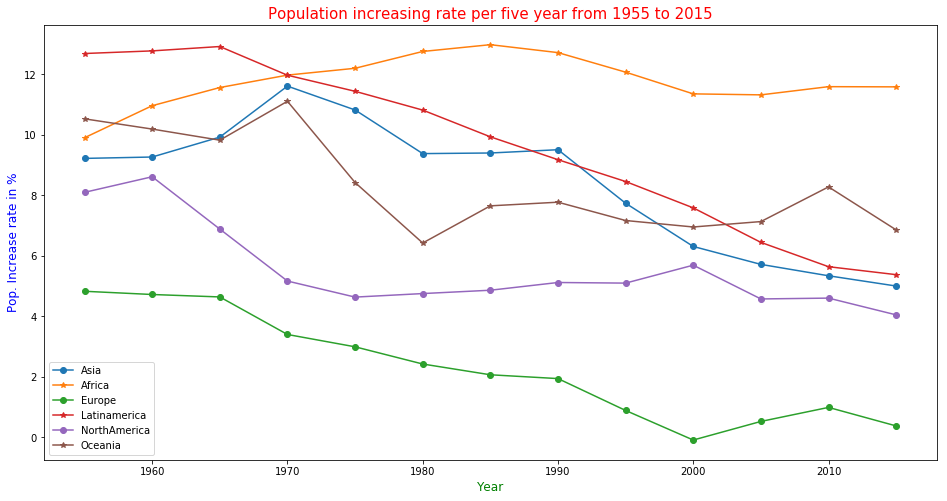This graph plot is about the population growth rate per five years of continents. The population growth rate in Europe is decreasing over the year slowly. The population increase rate is high for Africa and it will increase Africa’s population. Though Oceania has the Smallest population it has the second highest increasing rate. The high population increase rate of Asia is slowing down it seems the effect of economic boobs in some Asia country.


"""for density of population in continents we are taking the area in km.Sq
in list according to the population increasing order"""

Area_km ={"Asia":43820000,"Africa":30370000,"Europe":10180000,"Latinamerica":17840000,"Northamerica":24490000,"Oceania":9008500}
def density(pop,area):
dense=[]
for pops in pop:
dense.append(pops/area)
return dense
Asia_den=density(Asia,Area_km["Asia"])
Africa_den=density(Africa,Area_km["Africa"])
Europe_den=density(Europe,Area_km["Europe"])
LatinAmerica_den=density(Latinamerica,Area_km["Latinamerica"])
NorthAmerica_den=density(NorthAmerica,Area_km["Northamerica"])
Oceania_den=density(Oceania,Area_km["Oceania"])

In the following code, we are plotting a bar diagram with 6 multiple data. In the plt.bar() I used the year as X-axis and to print Six data in that one x-axis point it is needed to move the bar so all 6 bar can be shown. To show all bar I added 0.6 to every bar to print the bar in a new position so it will disturb other bars.


plt.figure(figsize=(18,9))# size of plotting field
X = np.arange(1950,2020,5)
plt.bar(X + 0.0, Asia_den, color = 'blue', width = 0.6)
plt.bar(X + 0.6, Africa_den, color = 'brown', width = 0.6)
plt.bar(X + 1.2, Europe_den, color = 'orange', width = 0.6)
plt.bar(X + 1.8, LatinAmerica_den, color = 'green', width = 0.6)
plt.bar(X + 2.4, NorthAmerica_den, color = 'gold', width = 0.6)
plt.bar(X + 3, Oceania_den, color = 'red', width = 0.6)
labels='Asia','Africa','Europe','LatinAmerica','NorthAmerica','Oceania'
plt.title("Population Density in Continents",size=15,color="red")
plt.xlabel("Years",size=12,color="green")
plt.ylabel("Thousand/KM.Sq",size=12,color="blue")
plt.legend(labels, loc="best")
plt.show()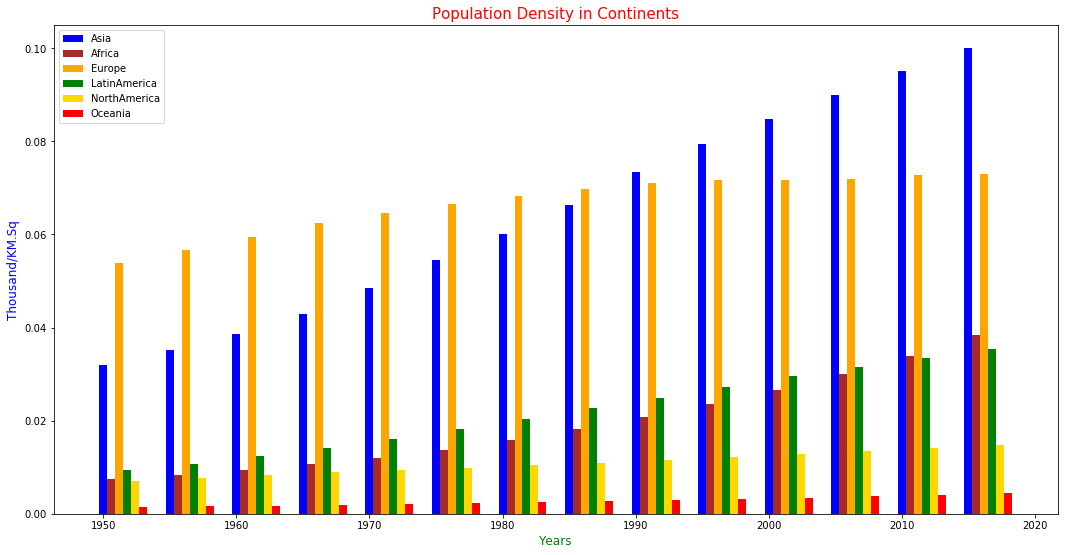In the above plot, we can see the density of the population over a period of time in Continents. In today’s time, the dense continent is Asia it left Europe behind in 1990 and Europe is 2nd dense continent. Oceania is the least dense country as its land area is big compared to Europe but the population is very small.


plt.figure(figsize=(14,7))
plt.title("Population of Earth from 1950 to 2015",size=15,color="red")
plt.xlabel("Years",size = 12,color="green")
plt.ylabel("Population in Thousand ",size=12,color="blue")
ploting(year,World,"o")
plt.show()

plt.show()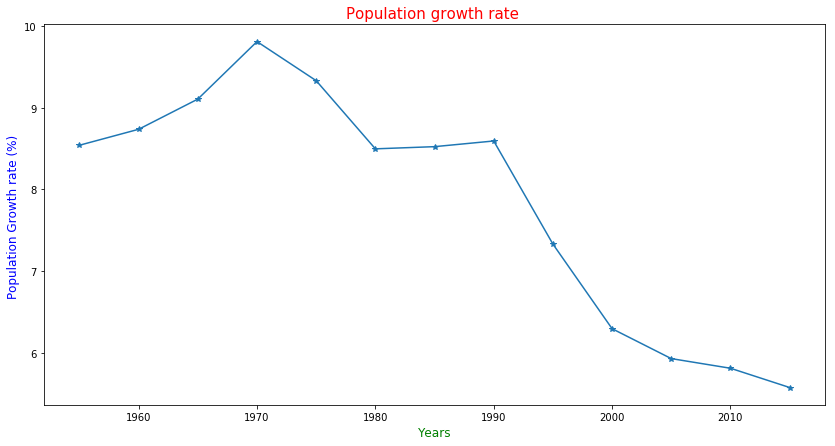The above plot shows the population Growth rate of the world on the basis of 5 years. This plot shows the Population growth rate from 1955 to 2015. The Growth rate started to decrease from 1970 when the rate was 9.80856% and it is 5.5783% for 2015. Though it seems rate is decreasing but the growth of population is fast as Population is large small growth rate also add a large number of new people to the population.